The STDIZE Procedure
 Computing Quantiles

PROC STDIZE offers two methods for computing quantiles: the one-pass approach and the order-statistics approach (like that used in the UNIVARIATE procedure).

The one-pass approach used in PROC STDIZE modifies the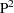algorithm for histograms proposed by Jain and Chlamtac (1985). The primary difference comes from the movement of markers. The one-pass method allows a marker to move to the right (or left) by more than one position (to the largest possible integer) as long as it does not result in two markers being in the same position. The modification is necessary in order to incorporate the FREQ variable.

You might obtain inaccurate results if you use the one-pass approach to estimate quantiles beyond the quartiles (that is, when you estimate quantiles < P25 or > P75). A large sample size (10,000 or more) is often required if the tail quantiles (quantiles <= P10 or >= P90 ) are requested. Note that, for variables with highly skewed or heavy-tailed distributions, tail quantile estimates might be inaccurate.

The order-statistics approach for estimating quantiles is faster than the one-pass method but requires that the entire data set be stored in memory. The accuracy in estimating the quantiles is comparable for both methods when the requested percentiles are between the lower and upper quartiles. The default is PCTLMTD=ORD_STAT if enough memory is available; otherwise, PCTLMTD=ONEPASS.

#### Computational Methods for the PCTLDEF= Option

You can specify one of five methods for computing quantile statistics when you use the order-statistics approach (PCTLMTD=ORD_STAT); otherwise, the PCTLDEF=5 method is used when you use the one-pass approach (PCTLMTD=ONEPASS).

##### Percentile Definitions

Letbe the number of nonmissing values for a variable, and let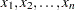represent the ordered values of the variable. For theth percentile, let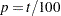. In the following definitions numbered 1, 2, 3, and 5, let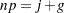whereis the integer part andis the fractional part of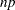. For definition 4, let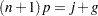Given the preceding definitions, theth percentile,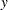, is defined as follows:

PCTLDEF=1

weighted average at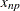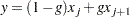where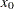is taken to be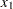PCTLDEF=2

observation numbered closest to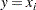whereis the integer part of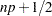if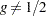. If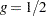, then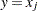ifis even, or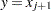ifis odd

PCTLDEF=3

empirical distribution function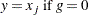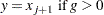PCTLDEF=4

weighted average aimed at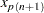where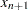is taken to be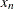PCTLDEF=5

empirical distribution function with averaging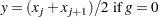#### Weighted Percentiles

When you specify a WEIGHT statement, or specify the NOTRUNCATE option in a FREQ statement, the percentiles are computed differently. The 100th weighted percentileis computed from the empirical distribution function with averaging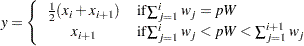where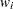is the weight associated with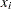, and where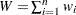is the sum of the weights.

For PCTLMTD= ORD_STAT, the PCTLDEF= option is not applicable when a WEIGHT statement is used, or when a NOTRUNCATE option is specified in a FREQ statement. However, in this case, if all the weights are identical, the weighted percentiles are the same as the percentiles that would be computed without a WEIGHT statement and with PCTLDEF=5.

For PCTLMTD= ONEPASS, the quantile computation currently does not use any weights.Previous Page | Next Page | Top of Page